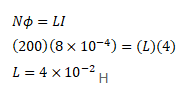# A magnetic flux of

Question:

A magnetic flux of $8 \times 10^{-4}$ weber is linked with each other of a 200 turn coil when there is an electric current of $4 \mathrm{~A}$ in it. Calculate the self-inductance of the coil.

Solution: